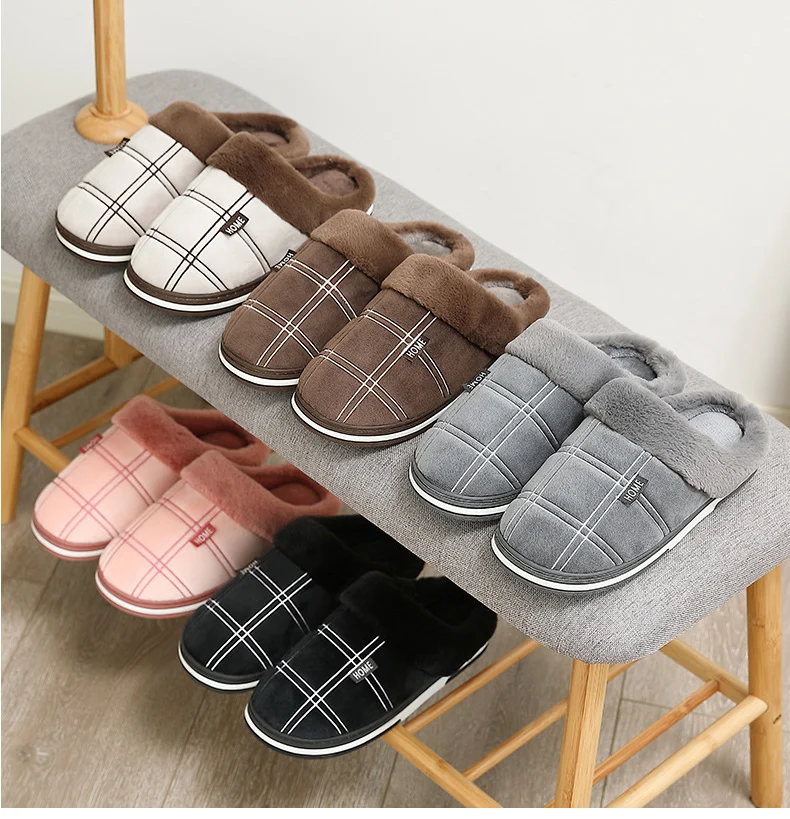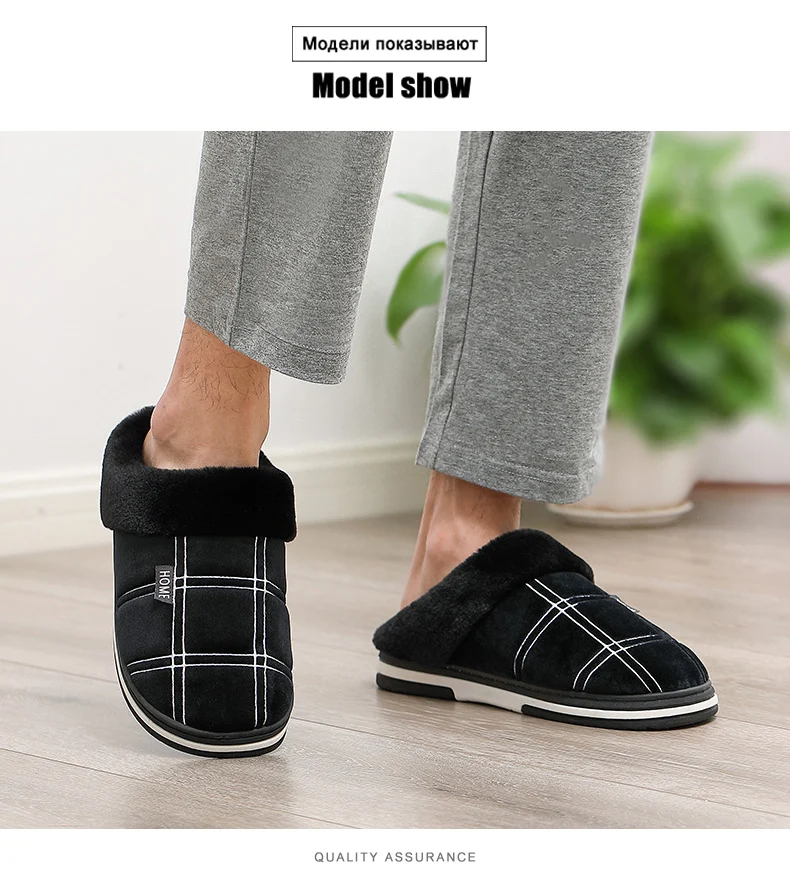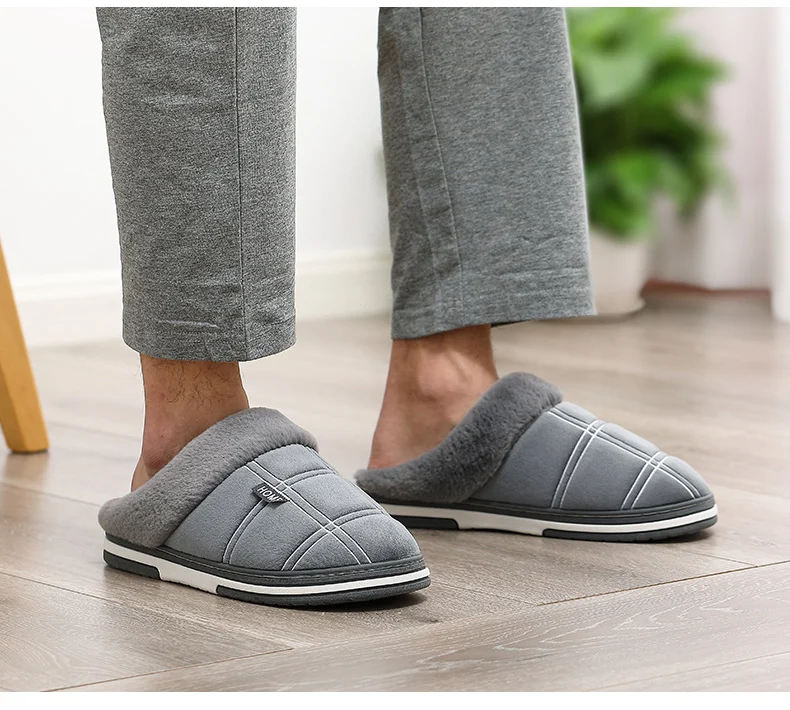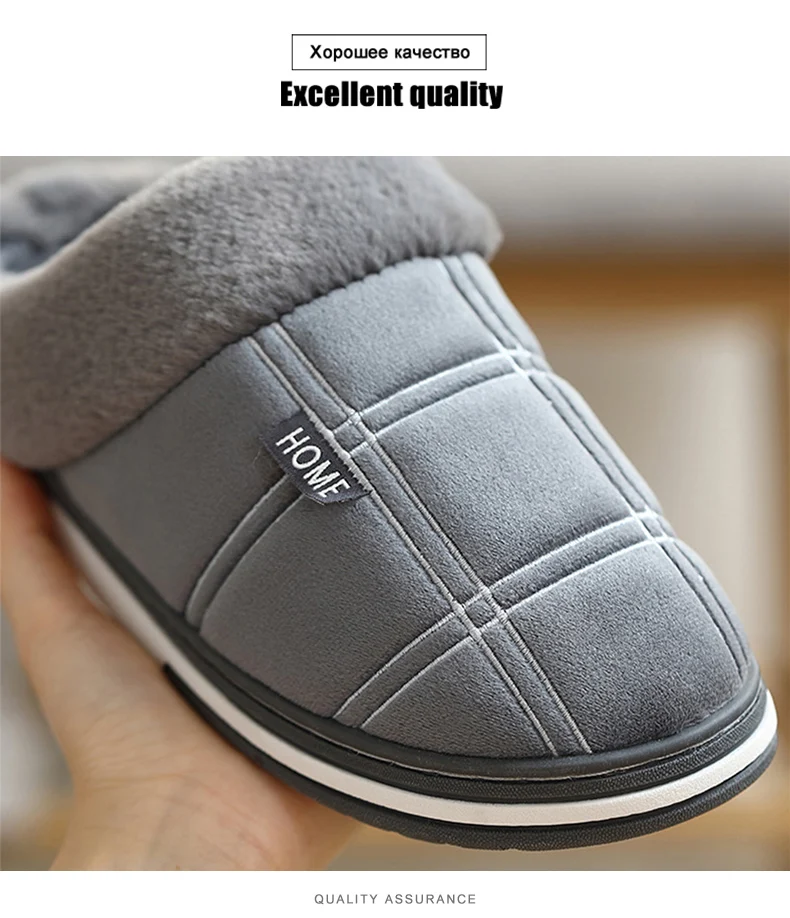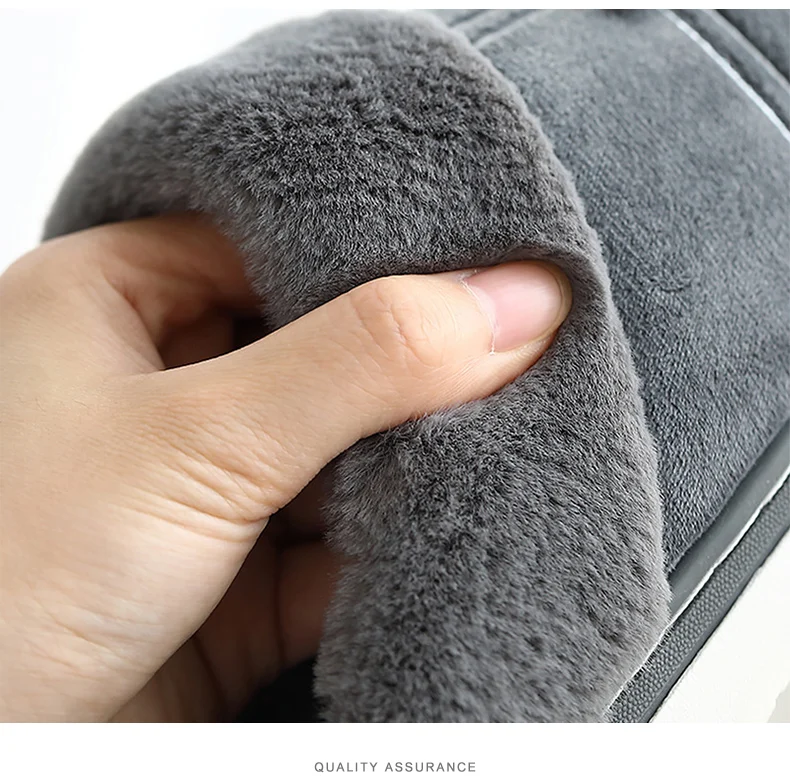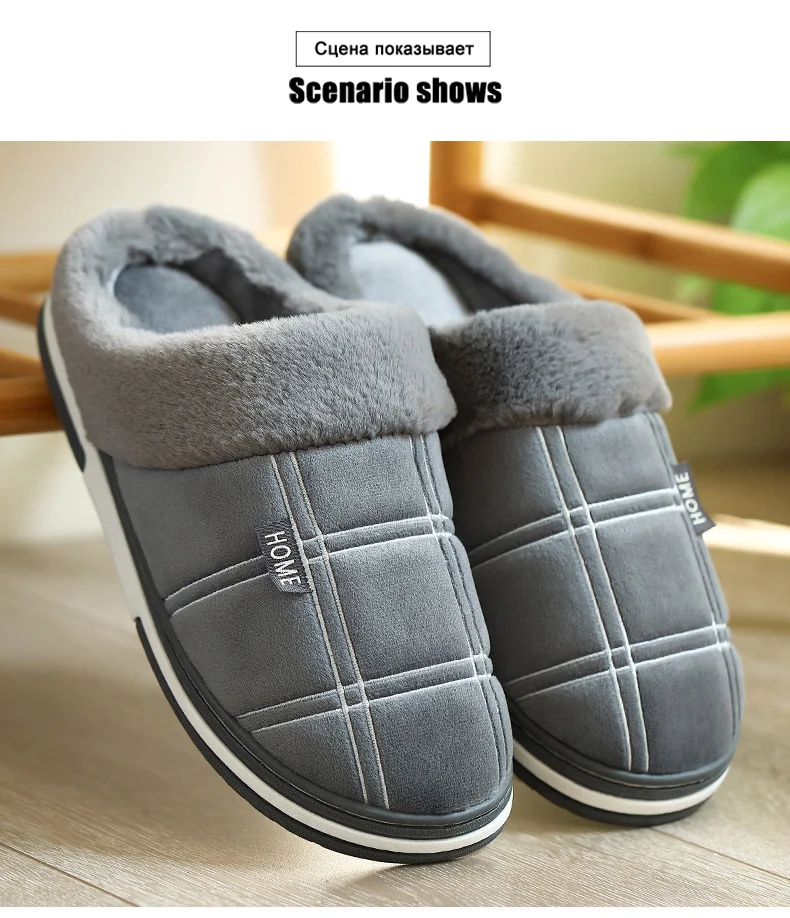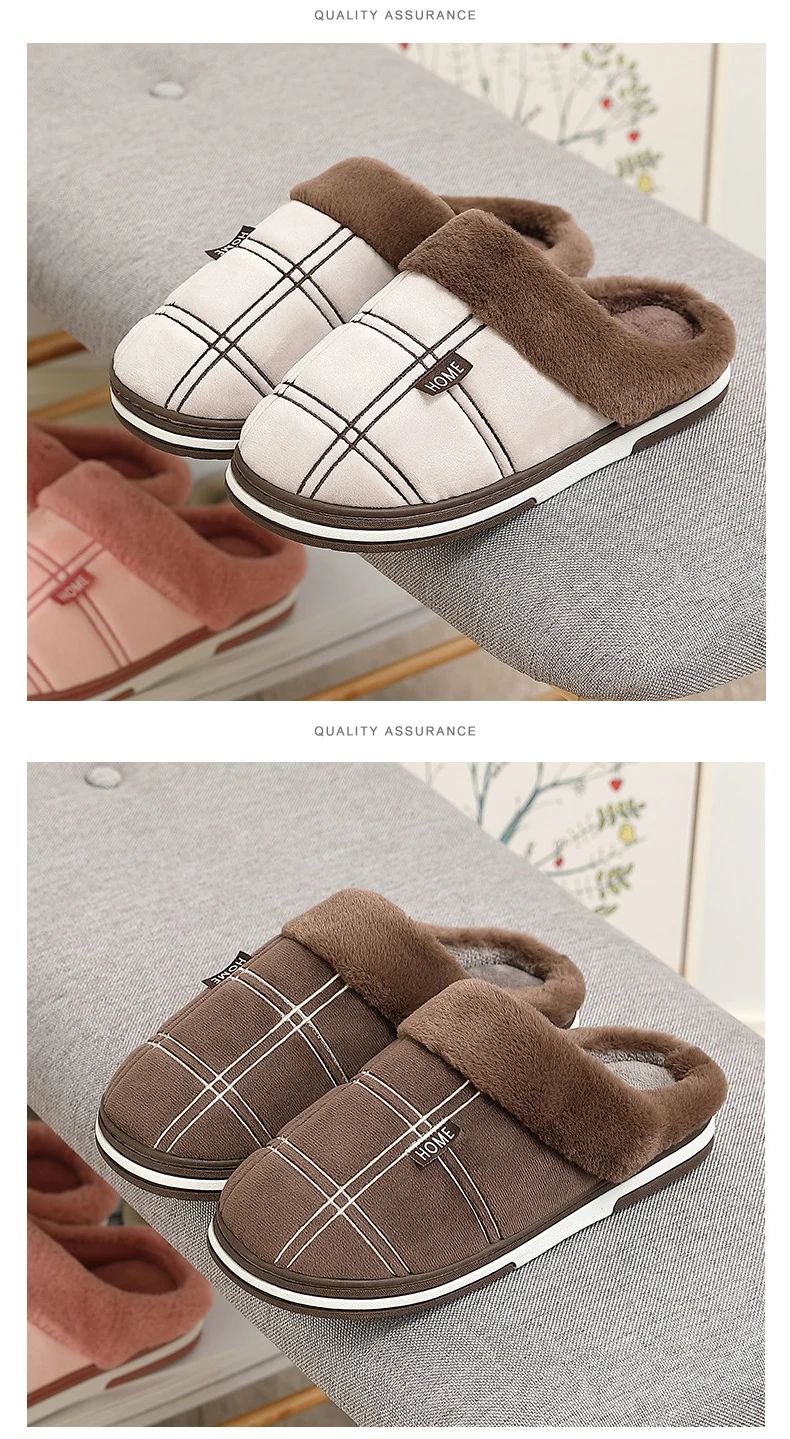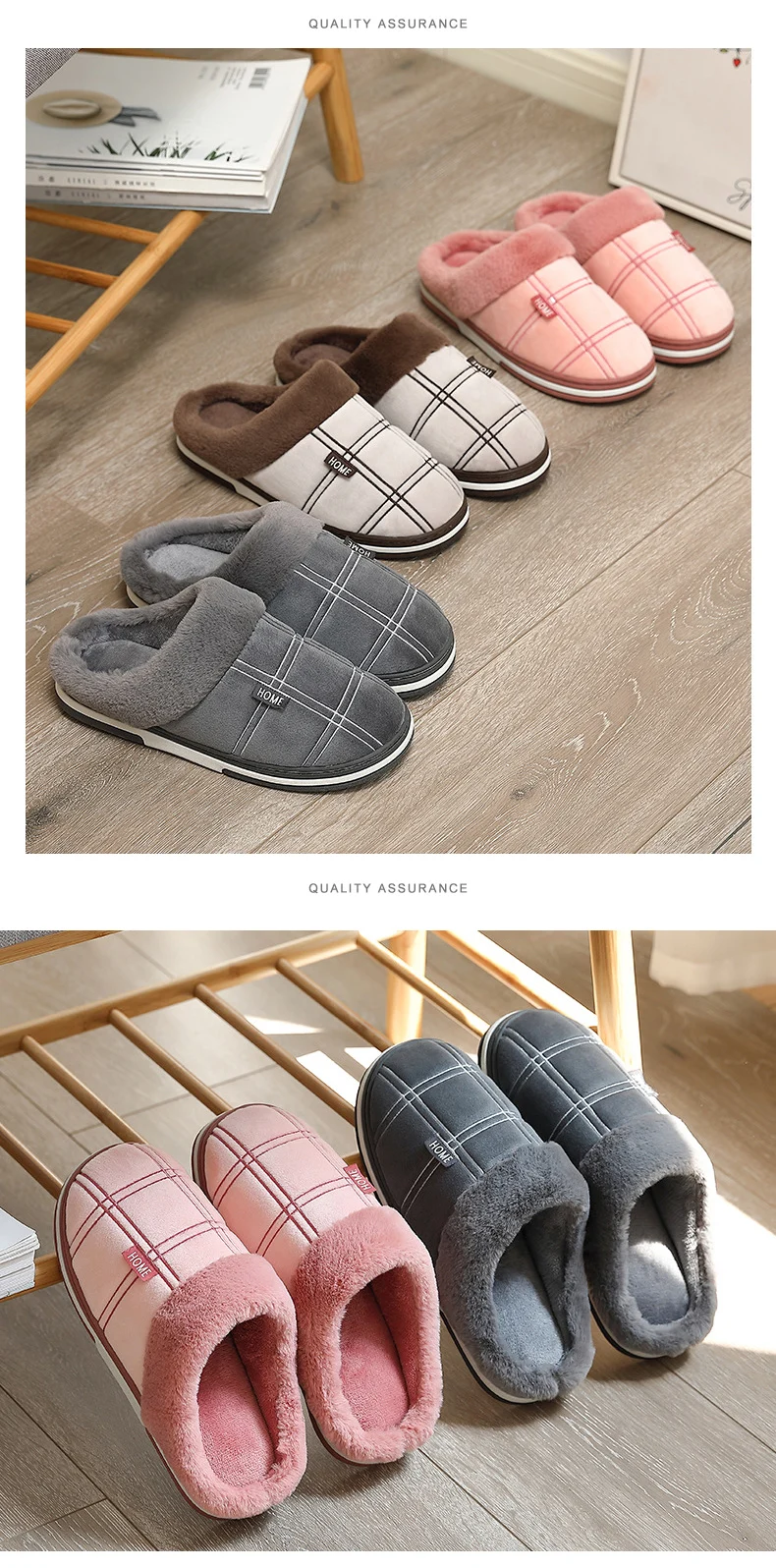# Winter home Men slippers Comfortable Soft Cotton slippers Men indoor Striped lattice plush Warm home house slipper Male

• \$28.27
Unit price per
• Save \$8.67
Tax included. Shipping calculated at checkout.

# Describe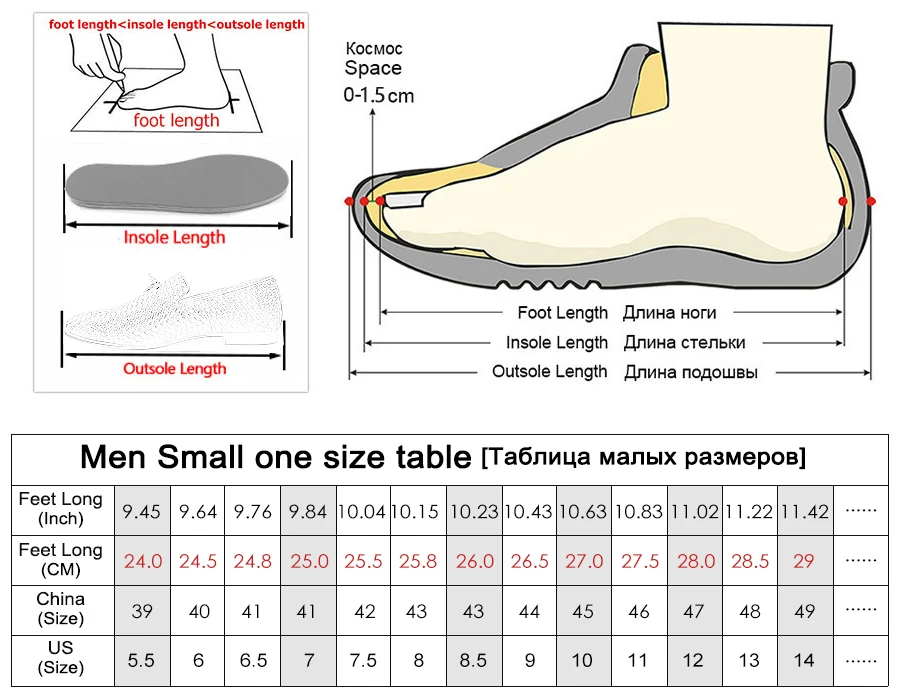Size Note：

Choose your size according to your feet length, For slippers, if you want to loose a little, you can choose a big one size, it is also very comfortable.

US size 4 = size 36/37=23.0 CM,
US size 4.5 = size 38/39=23.5 CM,
US size 5.5 =size 38/39=24.0 CM,
US size 6 = size 40/41=24.5 CM,
US size 7 = size 40/41=25.0 CM,
US size 7.5 = size 42/43=25.5 CM,
US size 8.5 = size 42/43=26.0 CM,
US size 9 = size 44/45=26.5 CM,
US size 10 = size 44/45=27.0 CM,
US size 11 = size 46/47=27.5 CM,
US size 12 = size 46/47=28.0 CM,
US size 13 = size 48/49=28.5 CM,
US size 14 = size 48/49=29.0 CM,
US size 15 = size 50/51=29.5 CM,
US size 16 = size 50/51=30.0 CM,